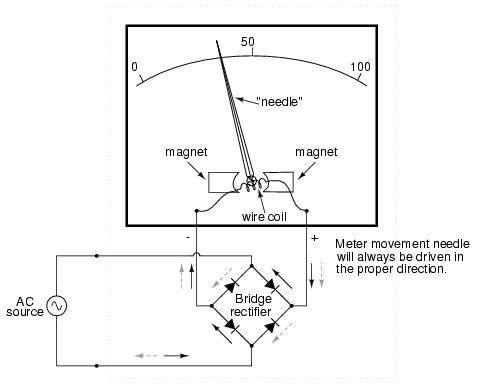# Selecting Electromechanical indicating instruments for current, voltage and power measurement

Types of instruments used for the measurement of current and voltage are as follows

No Instrument Suitable for
1 PMMC (Permanent magnet moving coil ) DC current
2 Moving iron type AC & DC
3 Electrodynamometer type AC & DC
4 Electrothermic AC & DC
5 Electrostatic AC & DC
6 Rectifier type AC & DC

# Sources of error in instruments

• Friction

• Heating of the instruments

• Expansion of the spring

• Lack of balance in the moving system

# PMMC

• Used for measurement of DC only

• In voltmeter, moving coil is mounted on metallic frame to provide electromagnetic damping

• In ammeter, moving coil is wound on non-magnetic former because the electromagnetic damping is provided by coil of the shunt.

• Material used for magnet in PMMC is AlNiCo ( Aluminium – Nickel – Cobalt )

• The field strength in PMMC varies from 0.1 – 1 Weber/m2

• Concentric magnetic construction is used to get longer angular movement of the pointer

• Angular displacement can be over 300 degrees

• Controlling forces in PMMC is provided by springs of phosphor bronze

• These fine wire springs are used for carrying coil current. So PMMC can be used only for low current and voltage measurement.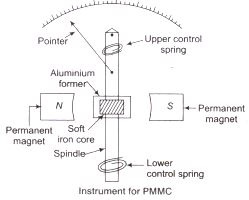## Forces acting in PMMC

Deflecting torque - Electromagnetic field

Controlling torque - Spring control .

Since at steady state for indication, both torques are in equilibrium , deflecting angle θ is α to current I, Magnetic field given by NBA

Therefore, aging of the spring affects the reading as well as decreasing magnetic field will gibe less reading.

Damping torque – Eddy current damping ( Voltmeter) , Electromagnetic damping (Ammeter)

## Sources of error in PMMC

1. Magnet aging and temperature

2. spring ageing and temperature

3. Change in resistance of coil with temperature

• Torque to weight ratios is high

• Highly sensitive

• Losses are minimum (25 – 200 𝞵w)

• High accuracy

• Single instrument can be used for multiple ranges

• Uniform scale

• High cost

• Measurement of DC only

# Moving iron

• In moving iron instruments, vane made up of soft iron and high permeablility steel is the moving element of the system

• It is so situated that it can move in the magnetic field of a stationary coil carrying current. The iron vane always try to adjust along the path of minimum reluctance.

• The magnetic field of the coil is 0.006 – 0.007 Weber/m2

## Forces acting in Moving iron

Deflecting torque - Magnetic induction.

Controlling torque – Spring constant

At equilibrium:

Deflection θ is α to square of current I, making the scale non-linear

Damping torque – Air friction

Since the magnetic field of the system is small, eddy current damping cannot be used as the eddy current field may distort the fields from the coil

## Types of moving coil instruments

• Attraction type - only one vane is moving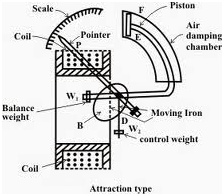• Repulsion type – There are two vanes, one movable and one fixed.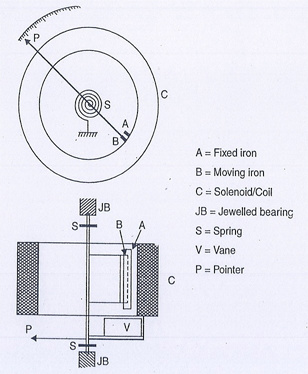The direction of force on the vane is independent on the direction of current in the coil . So this can be used for both AC and DC measurement.

## Errors in Moving iron instruments

• Hysteresis : Due to the hysteresis effect of the iron vane, the reading of the meter can be different for different cycles of the current in the coil.
• Frequency error : current to the meter Im is given as V/Z where Z is the impedance as the result of inductance effect in the coil, which is dependent ton the frequency. Hence when frequency decreases the Im will decreases and since this is proportional to deflection θ, for the same voltage, the deflection will be less.
• Eddy current error : Eddy current is not a problem for DC input or low frequency inputs up to 125 Hz , so moving iron can be used only till 125 Hz.

Calibration for AC and DC is different for the moving iron instruments

## Advantages of Moving iron instruments:

• Friction error is less

• Cheap and robust

• Used in aircrafts because they provide self-shielding to magnetic fields

## Disadvantages of Moving iron instruments

• Accuracy is around 0.2 tp 0.3 % at 50 Hz for a high grade meter

• Scale is non linear camped at the lower end of scale affecting accurate reading

• Errors due to hysteresis, frequency and stray magnetic fields are high.

• Sensitivity is less.

# Electrodynamometer type

• Both moving coil and fixed coil are connected and same current flows through them .

• Fixed coil is divided into two equal halves for uniform magnetic field.

• For milliammeter and voltmeter, the fixed coil is made of fine wire and for wattmeter and ammeter the fixed coil is heavy wire

• The movable coil is mounted on nonmagnetic former

• The magnetic fields in the coil have the is similar to moving iron instruments (0.005 – 0.006) Wb/m2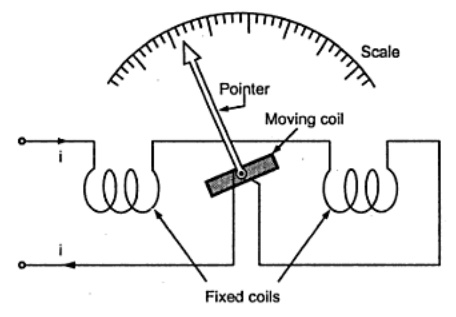## Forces acting on Electrodynamometer type:

Deflecting force – Mutual induction

Controlling force - Spring

At equilibrium, the deflection θ is α to square of the current (I) because the current in the coils are same and mutual induction force is the product of the current with change in mutual induction with respect to deflection.

The scale thus is non linear

Damping force - Air friction .

Eddy current will interfere with the coil magnetic field.

Irrespective of AC, or DC the deflection is same. So instruments calibrated for DC can also be used in AC measurement.

## Sources of error in electrodynamometer type

Eddy current error and Frequency error same as the MI.

## Advantages of electrodynamometer type instrument:

• Highly accurate.

• Frequency range can be up to 10kHz with appropriate static system to protect from external magnetic fields

• Effected by external magnetic field so will need a shielding with high permeability alloy which is the static system.

• Since mutual inductance is a cosine function of current when AC is the input, the deflection θ α I2 is only acceptable for -45⁰ to +45⁰ the angles where cosθ is 1.

• Sensitivity of the system is even less than moving iron.

# Electrothermic instruments

Used at higher frequencies they utilize hotwire, thermocouple or bolometer. These instruments are sued to read Irms and Vrms values,

### Hot wire

A wire heated by current passing through it. The thermoelectric hot wire is made of Platinum iridium.

Sensitivity is higher than electrodynamometer type.

### Thermocouple

• Measurement using the emf at the heated junction.

• These are used upto 500V and frequency more than 50Mz.

• Beyond this frequency there may be error due to skin effect which can be reduced using tubular conductor.

• This is preferable for current greater than 3A

• These are transfer instrument like the electrodynamometer meaning calibration can be done for DC and be used for AC measurement also.

• At low frequencies the voltmeter acts as precision instrument

• PMMC Is used for the detection of the thermocouple emf with its scale calibrated to measure RMS value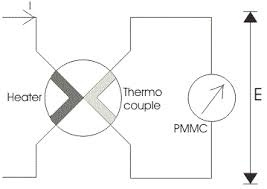### Bolometer :

Measure of the change in resistance due to heating caused by the current flowing through it.

• Not affected by magnetc field

• Not affected by frequency

• Can measure both AC and DC

# Electrostatic instrument

Use of attractive forces between two electrodes of opposite polarity. That is it uses electrostatic effect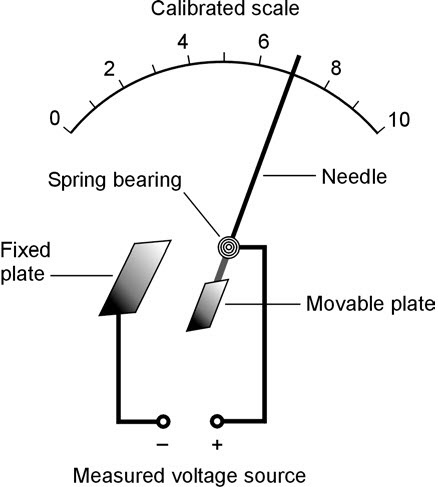These are voltage dependent hence the deflection is proportional to square voltage.

## Forces acting on electrostatic instrument:

Deflecting torque – Electrostatic attraction depending on the capacitance effect with parameters such as A of the electrode and space between them playing a major role.

Controlling torque- Spring

Since the deflection is proportional to the square of voltage and not dependent on the current, they can be used in systems were very less current need to be drawn from the system that is being measured. But the scale is non-linear

Damping torque - oil.

• Low power requirement

• No frequency error as heat generated is independent of frequency

• Stray magnetic field effect is absent

• Used I High voltage application

• Used for both AC and DC.

• Expensive

• Only used for high voltage measurement

• Non uniform scale

• Operating force is small.

# Rectifier type instruments

• Voltage to be measured is rectified before being detected by PMMC.

• These measure r.m.s value by calibrating the PMMC scale

• Used in communication or high current applications with maximum current approximation 1mA.

• Sensitivity is very high 1000 - 2000 Ω/V

• Rectification type instruments are of two types – Full wave rectification and half wave rectification.

• Rectification depends on the diode properties used as a unidirectional current flow device

• Materials used for diodes in this are Selenium, Germanium, Silicon.

• For power devices silicon is used because it is stable at high voltages